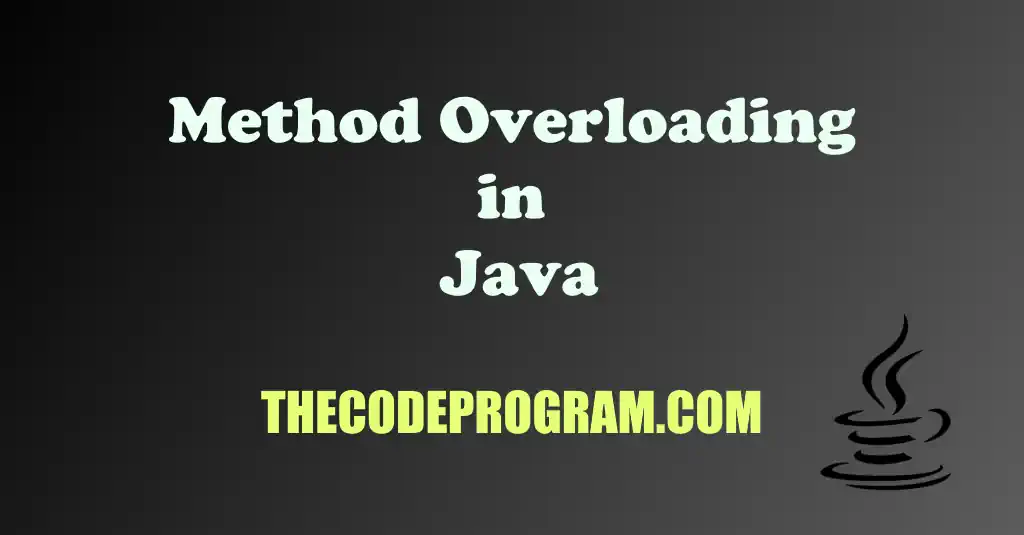Let's get started.

Overloading is redeclaring the functions with same name and different parameters. Method overloading is one of characteristics of object oriented programming. We can declare different types of parameters or different numbers of parameters.

Below you can see the example declaration syntax:
``````
void greet() {   }
void greet(String name) {   }
void greet(String name, String surname) {   }
void greet(UserInfo userInfo) {   }
``````

As you can see above ve overloaded the greet function. We declared different number of parameters and different types of parameters for same named function.

``````
public class Main {
public static void main(String[] args) {
greet();
greet("Burak");
greet("Burak Hamdi", "TUFAN");
}

public static void greet(){
System.out.println("No Parameter...");
}

public static void greet(String name){
System.out.println(name);
}

public static void greet(String name, String surname){
System.out.println(name + " " + surname );
}
}
``````
Program output will be like below:
``````
No Parameter...
Burak
Burak Hamdi TUFAN
``````

Below you can see another example. This example will demonstrate the method overloading over constructors. In this example you will see a prototype numeric wrapper class like BigDecimal. You can initialise the number class with double, integer and string with constructor.

``````
public class Main {
public static void main(String[] args) {
NumHandler nh1 = new NumHandler(10);
nh1.printNumber();
NumHandler nh2 = new NumHandler(30.75);
nh2.printNumber();
NumHandler nh3 = new NumHandler("150");
nh3.printNumber();
}
}

public class NumHandler{
int number;

public NumHandler(int n){ this.number = n; }
public NumHandler(double n){ this.number = (int)n; }
public NumHandler(String n){ this.number = Integer.parseInt(n); }

public void printNumber(){
System.out.println(this.number);
}
}
``````
Program output will be like below:
``````
10
30
150
``````

With method overloading we can use same method name for different type of operations instead of creating different functions for all of different logic. In here important point is we need to change parameter types and number of parameters. If we change the return type of method, it is not method overloading.

Burak Hamdi TUFAN

19/08/2015

18/03/2021

02/04/2021